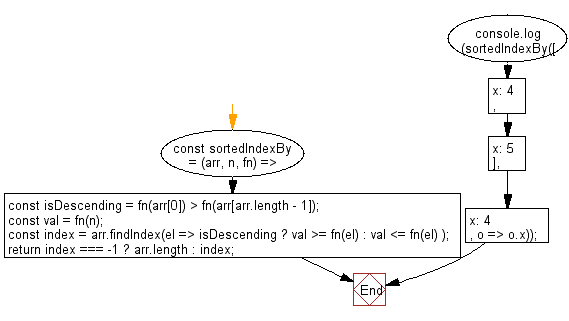# JavaScript Sorting Algorithm: Sorted IndexBy

## JavaScript Sorting Algorithm: Exercise-20 with Solution

Write a JavaScript program to find the lowest index at which a value should be inserted into an array to maintain its sorting order. This is based on the provided iterator function.

• Loosely check if the array is sorted in descending order.
• Use Array.prototype.findIndex() to find the appropriate index where the element should be inserted, based on the iterator function fn.

Sample Solution:

JavaScript Code:

``````const sortedIndexBy = (arr, n, fn) => {
const isDescending = fn(arr) > fn(arr[arr.length - 1]);
const val = fn(n);
const index = arr.findIndex(el =>
isDescending ? val >= fn(el) : val <= fn(el)
);
return index === -1 ? arr.length : index;
};

console.log(sortedIndexBy([{ x: 4 }, { x: 5 }], { x: 4 }, o => o.x));
```
```

Sample Output:

```0
```

Flowchart:Live Demo:

See the Pen javascript-common-editor by w3resource (@w3resource) on CodePen.

Improve this sample solution and post your code through Disqus

What is the difficulty level of this exercise?

Test your Programming skills with w3resource's quiz.

﻿